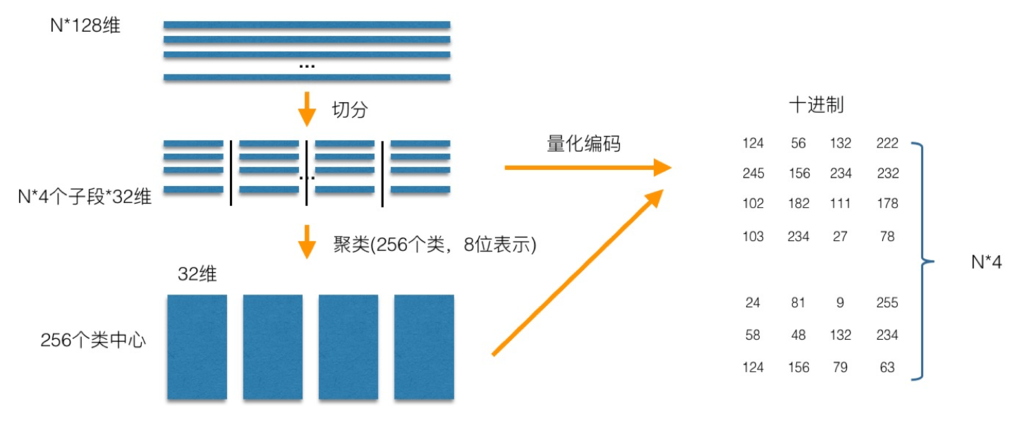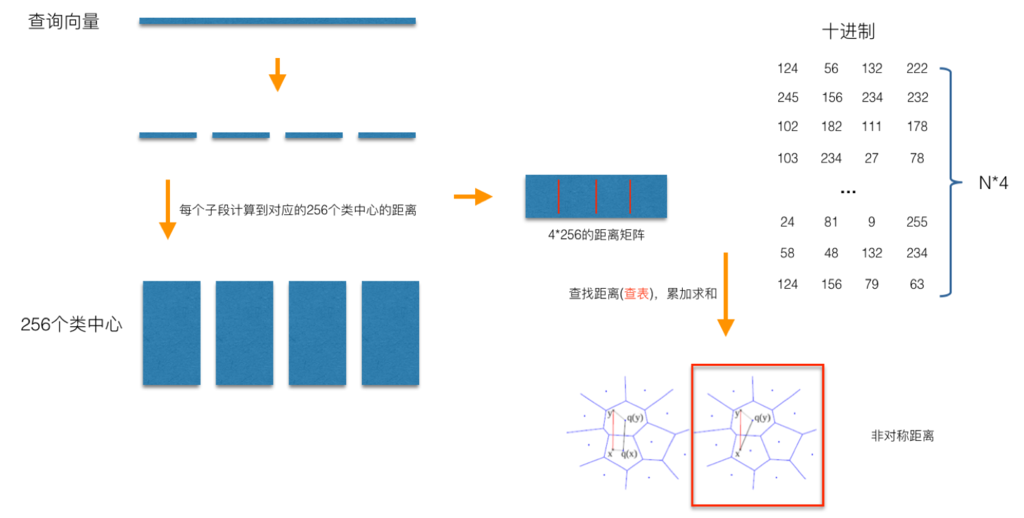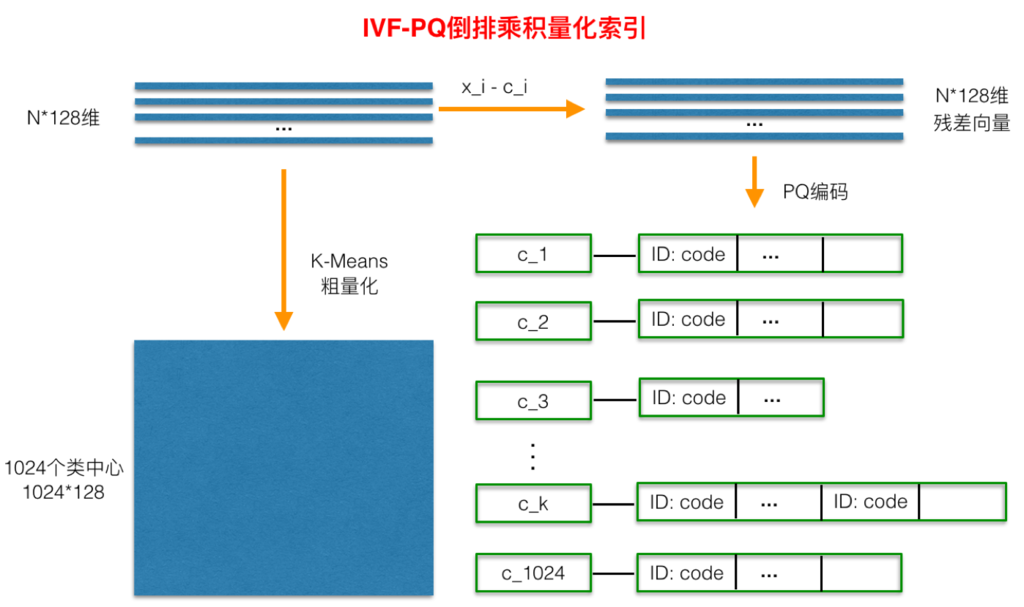# 【语音识别】基于矢量量化（VQ）的说话人识别matlab源码

• 时间:
• 浏览:

## 一、简介

1 PQ乘积量化
PQ乘积量化的核心思想还是聚类，或者说具体应用到ANN近似最近邻搜索上，K-Means是PQ乘积量化子空间数目为1的特例。PQ乘积量化生成码本和量化的过程可以用如下图示来说明：2 倒排乘积量化## 二、源代码

``````function mfc=my_mfcc(x,fs)
%MY_MFCC:获取Speaker recognition的参数
%x:输入语音信号  fs：采样率
%mfc：十二个mfcc系数和一个能量 以及一阶和二阶差分 共36个参数 每一行为一帧数据
%clc,clear
N=256;p=24;
bank=mel_banks(p,N,fs,0,fs/2);
% 归一化mel滤波器组系数
bank=full(bank);
bank=bank/max(bank(:));

% 归一化倒谱提升窗口
w = 1 + 6 * sin(pi * [1:12] ./ 12);
w = w/max(w);

% 预加重滤波器
x=double(x);
x=filter([1 -0.9375],1,x);

% 语音信号分帧
sf=check_ter(x,N,128,10);

x=div_frame(x,N,128);
%sf=sp_ter(x,4,16);
%m=zeros(size(x,1),13);
m=zeros(1,13);
% 计算每帧的MFCC参数
for i=1:size(x,1)
if sf(i)==1
% j=j+1;
y = x(i,:);
y = y' .* hamming(N);
energy=log(sum(y.^2)+eps);%能量
y = abs(fft(y));
y = y.^2+eps;
c1=dct(log(bank * y));
c2 = c1(2:13).*w';%取2~13个系数
%m(i,:)=[c2;energy]';
m1=[c2;energy]';
%m1=c2';
m=[m;m1];
end

end

%差分系数
dm = zeros(size(m));
dmm= zeros(size(m));
for i=2:size(m,1)-1
dm(i,:) = (m(i,:) - m(i-1,:));
end
for i=3:size(m,1)-2
dmm(i,:) = (dm(i,:) - dm(i-1,:));
end
%dm = dm / 3;
function v=lbg(x,k)
%lbg：完成lbg均值聚类算法
% lbg(x,k) 对输入样本x，分成k类。其中，x为row*col矩阵，每一列为一个样本，
% 每个样本有row个元素。
% [v1 v2 v3 ...vk]=lbg(...)返回k个分类，其中vi为结构体，vi.num为该类
% 中含有元素个数，vi.ele(i)为第i个元素值，vi.mea为相应类别的均值

[row,col]=size(x);
%u=zeros(row,k);%每一列为一个中心值
epision=0.03;%选择epision参数
delta=0.01;
%u2=zeros(row,k);
%LBG算法产生k个中心
u=mean(x,2);%第一个聚类中心，总体均值
for i3=1:log2(k)
u=[u*(1-epision),u*(1+epision)];%双倍
%time=0;
D=0;
DD=1;
while abs(D-DD)/DD>delta   %sum(abs(u2(:).^2-u(:).^2))>0.5&&(time<=80)   %u2~=u
DD=D;
for i=1:2^i3            %初始化
v(i).num=0;
v(i).ele=zeros(row,1);
end
for i=1:col %第i个样本
distance=dis(u,x(:,i));%第i个样本到各个中心的距离
[val,pos]=min(distance);
v(pos).num=v(pos).num+1;%元素的数量加1
if v(pos).num==1    %ele为空
v(pos).ele=x(:,i);
else
v(pos).ele=[v(pos).ele,x(:,i)];
end
end
for i=1:2^i3
u(:,i)=mean(v(i).ele,2);%新的均值中心
for m=1:size(v(i).ele,2)
D=D+sum((v(i).ele(m)-u(:,i)).^2);
end
end
end
end
``````

## 三、运行结果## 四、备注

### 往期回顾>>>>>>

【语音识别】基于隐马尔可夫模型（HMM）的孤立字语音识别matlab源码

【信号处理】基于HMM的睡眠状态检测matlab源码

【信号处理】基于小波变换的音频水印嵌入提取matlab源码

【信号处理】基于遗传算法的VST混响matlab源码

【信号处理】脉搏信号之脉率存档matlab源码含GUI

【信号处理】单通道盲源分离（SSA-ICA）算法

【信号处理】数字电子琴设计与实现matlab源码

【信号处理】LDPC码的校验矩阵、编译码matlab源码

【语音加密】基于混沌算法的语音信号加密解密matlab源码

【信号处理】基于小波变换的语音增强matlab源码

【语音增强】基于维纳滤波之语音增强matlab源码
【信号处理】OFDM-MIMO通信建模与仿真matlab源码

【信号处理】数字调制信号仿真matlab源码含GUI

【信号处理】基于LMS算法信号去噪matlab源码

【信号处理】基于小波变换的量化音频数字水印matlab源码

【信号处理】基于小波变换的音频水印嵌入与提取matlab源码

【语音识别】基于BP神经网络的语音情感识别matlab源码

【信号处理】基于小波变换的音频水印嵌入与提取matlab源码

【情感识别】基于改进KNN语音情感分类识别malab源码含GUI

【信号去噪】基于谱减法去噪matlab源码